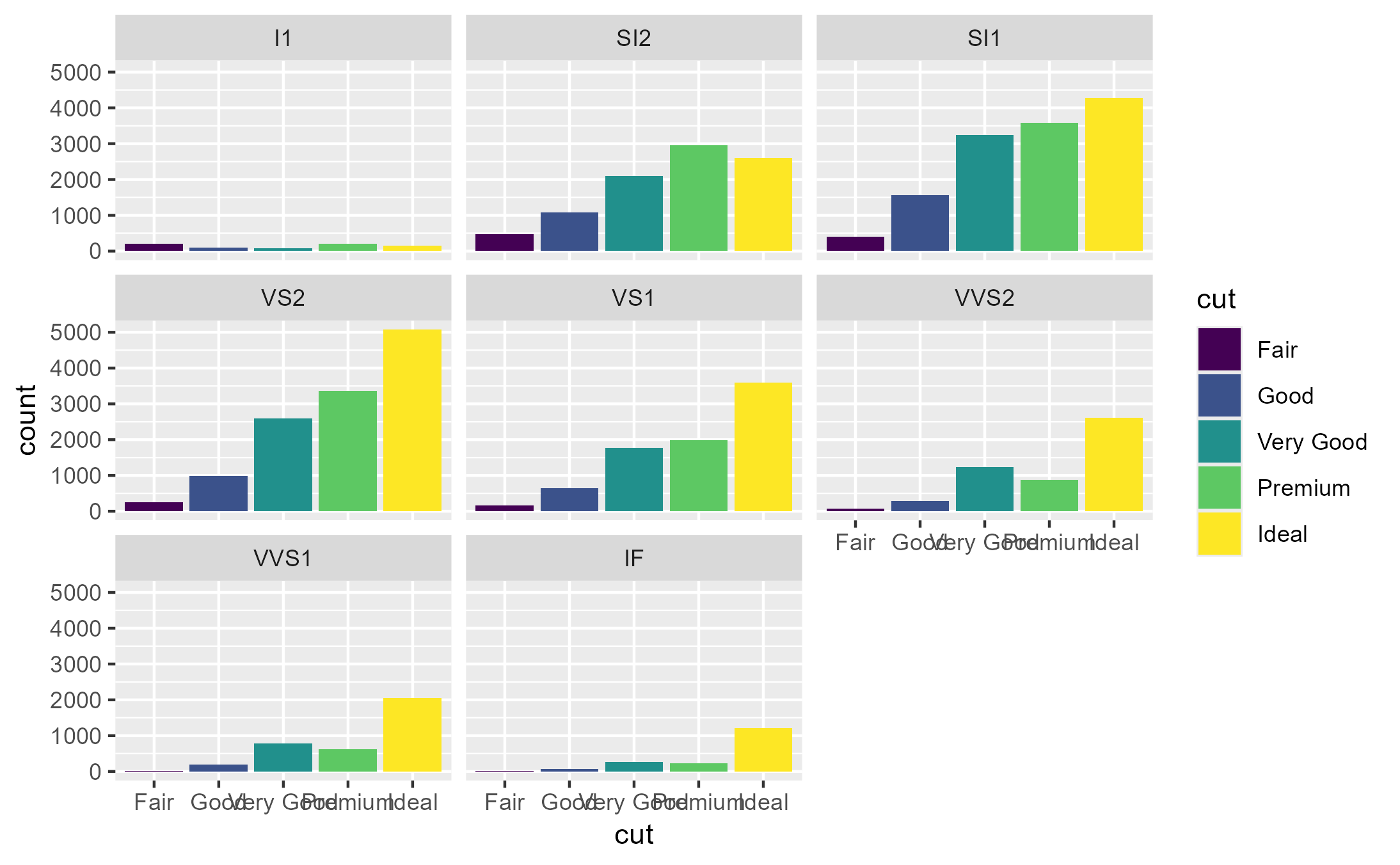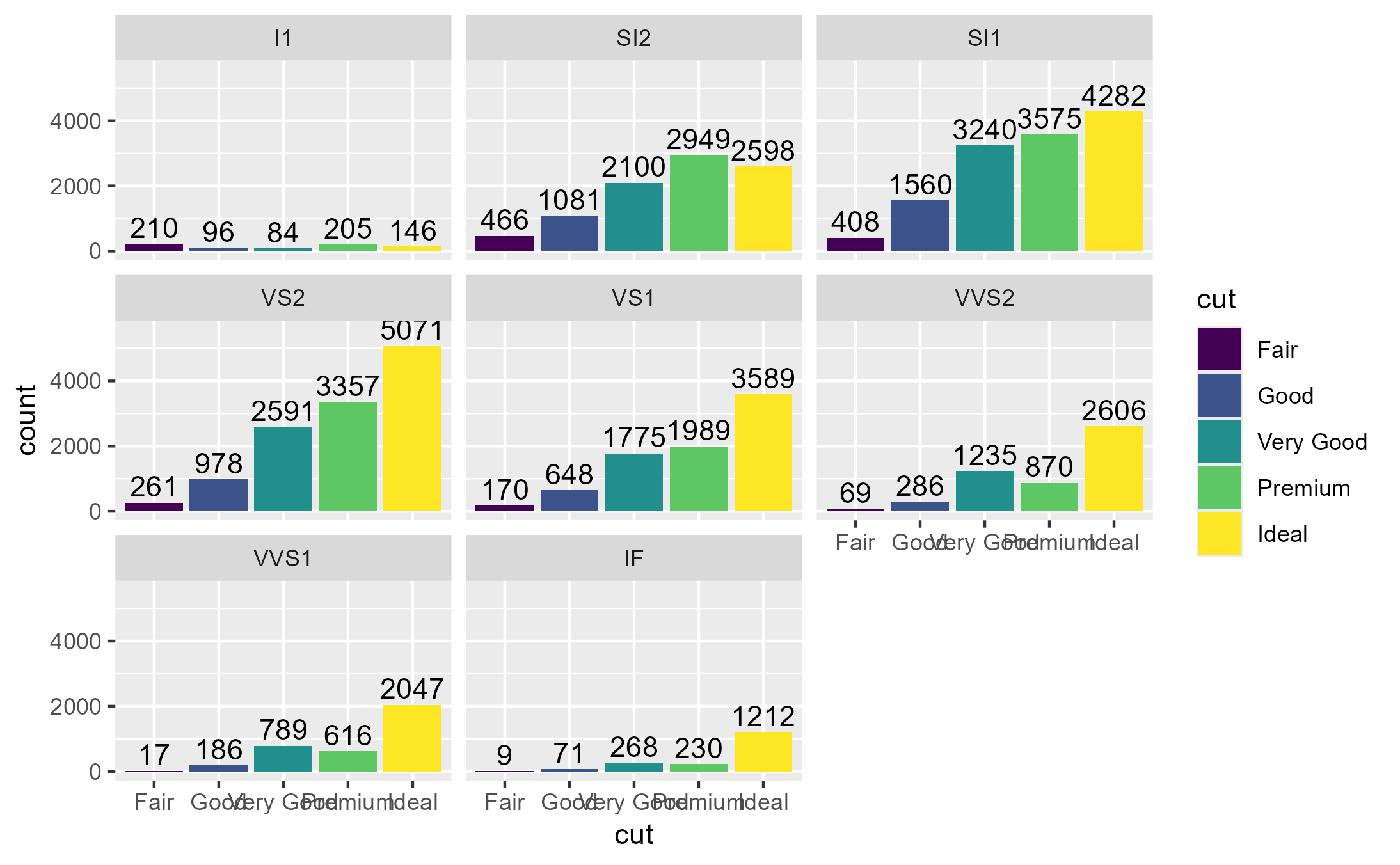Inspect how many times a method was called

## Usage

ggtrace_inspect_n(x, method, error = FALSE)

## Arguments

x

A ggplot object

method

A function or a ggproto method. The ggproto method may be specified using any of the following forms:

• ggproto$method • namespace::ggproto$method

• namespace:::ggproto$method error If TRUE, continues inspecting the method until the ggplot errors. This is useful for debugging but note that it can sometimes return incomplete output. ## Value The number of times method was called in the evaluation of x ## Examples  library(ggplot2) p1 <- ggplot(diamonds, aes(cut)) + geom_bar(aes(fill = cut)) + facet_wrap(~ clarity) p1# 1 call to Stat$compute_layer
ggtrace_inspect_n(p1, Stat$compute_layer) #>  1 # 8 calls to Stat$compute_panel
ggtrace_inspect_n(p1, Stat$compute_panel) #>  8 # Note that there are 0 calls to Stat$compute_group ...
ggtrace_inspect_n(p1, Stat$compute_group) #>  0 # because StatCount has its own "compute_group" method defined ggtrace_inspect_n(p1, StatCount$compute_group)
#>  40

# How about if we add a second layer that uses StatCount?
p2 <- p1 + geom_text(
aes(label = after_stat(count)),
stat = StatCount, position = position_nudge(y = 500)
)

p2# Now there are double the calls to Stat/StatCount methods
ggtrace_inspect_n(p2, Stat$compute_layer) #>  2 ggtrace_inspect_n(p2, Stat$compute_panel)
#>  16
ggtrace_inspect_n(p2, StatCount$compute_group) #>  80 # But separate calls to each layer's respective Geoms ggtrace_inspect_n(p2, GeomBar$draw_panel)
#>  8
ggtrace_inspect_n(p2, GeomText\$draw_panel)
#>  8